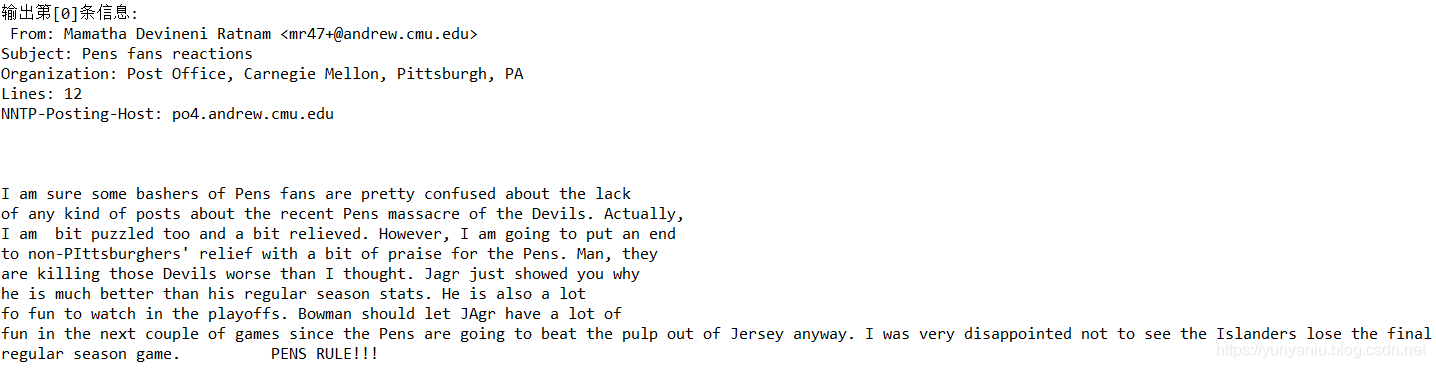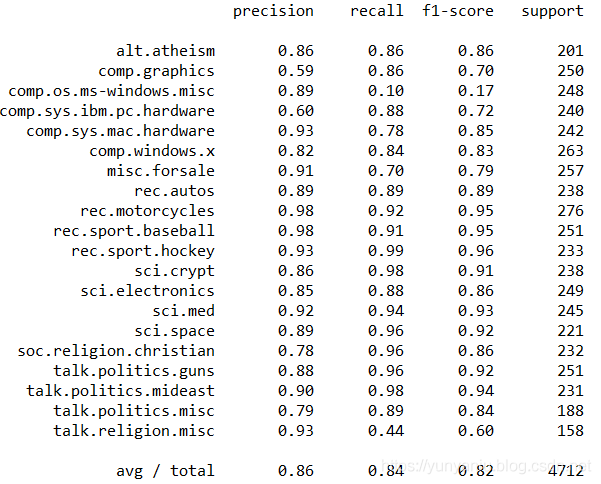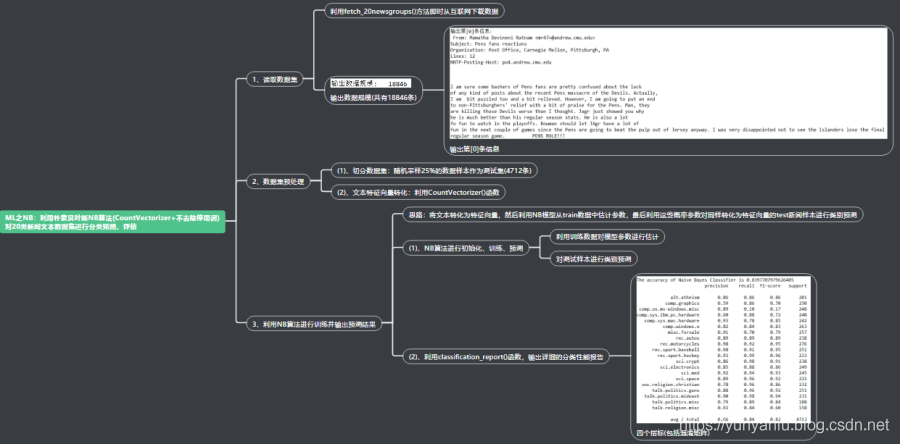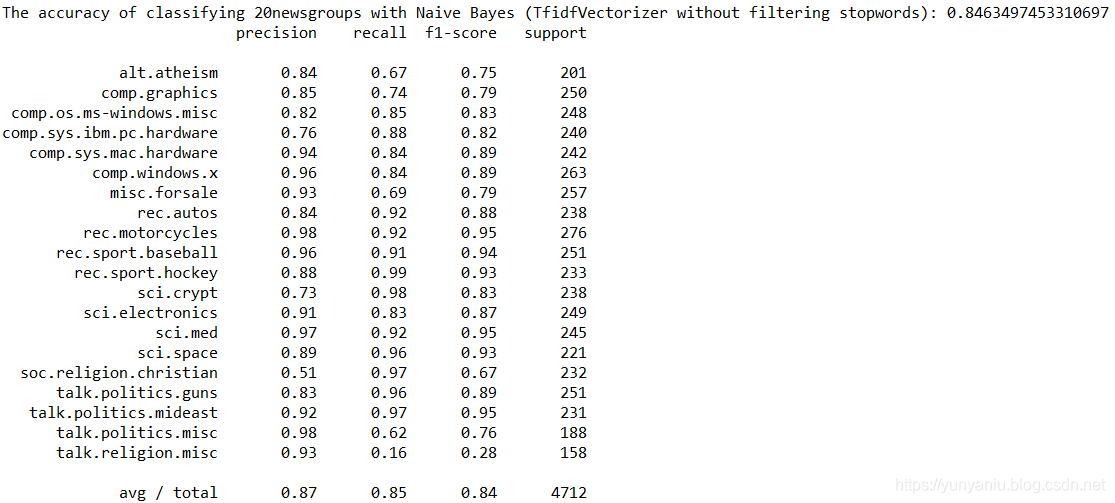# ML之NB：利用朴素贝叶斯NB算法(CountVectorizer+不去除停用词)对fetch_20newsgroups数据集(20类新闻文本)进行分类预测、评估

+关注继续查看

## 输出结果## 设计思路## 核心代码

class MultinomialNB Found at: sklearn.naive_bayes

class MultinomialNB(BaseDiscreteNB):

"""

Naive Bayes classifier for multinomial models

The multinomial Naive Bayes classifier is suitable for classification with

discrete features (e.g., word counts for text classification). The

multinomial distribution normally requires integer feature counts. However,

in practice, fractional counts such as tf-idf may also work.

Read more in the :ref:User Guide <multinomial_naive_bayes>.

Parameters

----------

alpha : float, optional (default=1.0)

(0 for no smoothing).

fit_prior : boolean, optional (default=True)

Whether to learn class prior probabilities or not.

If false, a uniform prior will be used.

class_prior : array-like, size (n_classes,), optional (default=None)

Prior probabilities of the classes. If specified the priors are not

Attributes

----------

class_log_prior_ : array, shape (n_classes, )

Smoothed empirical log probability for each class.

intercept_ : property

Mirrors class_log_prior_ for interpreting MultinomialNB

as a linear model.

feature_log_prob_ : array, shape (n_classes, n_features)

Empirical log probability of features

given a class, P(x_i|y).

coef_ : property

Mirrors feature_log_prob_ for interpreting MultinomialNB

as a linear model.

class_count_ : array, shape (n_classes,)

Number of samples encountered for each class during fitting. This

value is weighted by the sample weight when provided.

feature_count_ : array, shape (n_classes, n_features)

Number of samples encountered for each (class, feature)

during fitting. This value is weighted by the sample weight when

provided.

Examples

--------

>>> import numpy as np

>>> X = np.random.randint(5, size=(6, 100))

>>> y = np.array([1, 2, 3, 4, 5, 6])

>>> from sklearn.naive_bayes import MultinomialNB

>>> clf = MultinomialNB()

>>> clf.fit(X, y)

MultinomialNB(alpha=1.0, class_prior=None, fit_prior=True)

>>> print(clf.predict(X[2:3]))



Notes

-----

For the rationale behind the names coef_ and intercept_, i.e.

naive Bayes as a linear classifier, see J. Rennie et al. (2003),

Tackling the poor assumptions of naive Bayes text classifiers, ICML.

References

----------

C.D. Manning, P. Raghavan and H. Schuetze (2008). Introduction to

Information Retrieval. Cambridge University Press, pp. 234-265.

classification-1.html

"""

def __init__(self, alpha=1.0, fit_prior=True, class_prior=None):

self.alpha = alpha

self.fit_prior = fit_prior

self.class_prior = class_prior

def _count(self, X, Y):

"""Count and smooth feature occurrences."""

if np.any((X.data if issparse(X) else X) < 0):

raise ValueError("Input X must be non-negative")

self.feature_count_ += safe_sparse_dot(Y.T, X)

self.class_count_ += Y.sum(axis=0)

def _update_feature_log_prob(self, alpha):

"""Apply smoothing to raw counts and recompute log probabilities"""

smoothed_fc = self.feature_count_ + alpha

smoothed_cc = smoothed_fc.sum(axis=1)

self.feature_log_prob_ = np.log(smoothed_fc) - np.log(smoothed_cc.

reshape(-1, 1))

def _joint_log_likelihood(self, X):

"""Calculate the posterior log probability of the samples X"""

check_is_fitted(self, "classes_")

X = check_array(X, accept_sparse='csr')

return safe_sparse_dot(X, self.feature_log_prob_.T) + self.class_log_prior_1314 01079 0ML之NB：利用朴素贝叶斯NB算法(CountVectorizer+不去除停用词)对fetch_20newsgroups数据集(20类新闻文本)进行分类预测、评估
ML之NB：利用朴素贝叶斯NB算法(CountVectorizer+不去除停用词)对fetch_20newsgroups数据集(20类新闻文本)进行分类预测、评估
84 01360 0931 02605 0⭐️STL⭐️之string和vector全解，❤️算法必备❤️＜上＞
⭐️STL⭐️之string和vector全解，❤️算法必备❤️＜上＞
36 01369 01074 0ML之NB：利用朴素贝叶斯NB算法(TfidfVectorizer+不去除停用词)对20类新闻文本数据集进行分类预测、评估
ML之NB：利用朴素贝叶斯NB算法(TfidfVectorizer+不去除停用词)对20类新闻文本数据集进行分类预测、评估
80 0

1702

0

JS零基础入门教程（上册）